1. /
2. CBSE
3. /
4. Class 06
5. /
6. Mathematics
7. /
8. NCERT Solutions for Class...

# NCERT Solutions for Class 6 Maths Exercise 14.5

NCERT solutions for Maths Practical geometry## NCERT Solutions for Class 6 Maths Practical geometry

###### Question 1.Draw {tex}\overline {{\text{AB}}} {/tex} of length 7.3 cm and find its axis of symmetry.

Answer: Axis of symmetry of line segment {tex}\overline {{\text{AB}}} {/tex} will be the perpendicular bisector of {tex}\overline {{\text{AB}}} .{/tex} So, draw the perpendicular bisector of AB.

Steps of construction: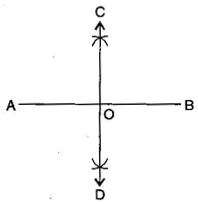(i) Draw a line segment {tex}\overline {{\text{AB}}} {/tex} = 7.3 cm

(ii) Taking A and B as centres and radius more than half of AB, draw two arcs which intersect each other at C and D.

(iii) Join CD. Then CD is the axis of symmetry of the line segment AB.

###### Question 2.Draw a line segment of length 9.5 cm and construct its perpendicular bisector.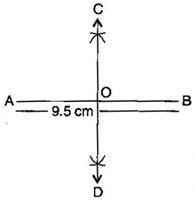(i) Draw a line segment {tex}\overline {{\text{AB}}} {/tex} = 9.5 cm

(ii) Taking A and B as centres and radius more than half of AB, draw two arcs which intersect each other at C and D.

(iii) Join CD. Then CD is the perpendicular bisector of {tex}\overline {{\text{AB}}} {/tex}.

NCERT Solutions for Class 6 Maths Exercise 14.5

###### Question 3.Draw the perpendicular bisector of {tex}\overline {{\text{XY}}} {/tex} whose length is 10.3 cm.

(a) Take any point P on the bisector drawn. Examine whether PX = PY.

(b) If M is the mid-point of {tex}\overline {{\text{XY}}} {/tex}, what can you say about the lengths MX and XY?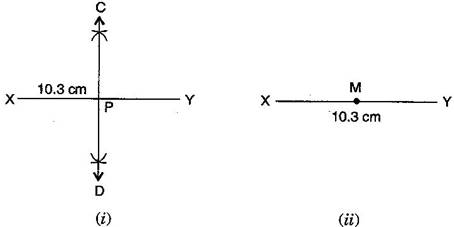(i) Draw a line segment {tex}\overline {{\text{XY}}} {/tex} = 10.3 cm

(ii) Taking X and Y as centres and radius more than half of AB, draw two arcs which intersect each other at C and D.

(iii) Join CD. Then CD is the required perpendicular bisector of {tex}\overline {{\text{XY}}} {/tex}.

Now:

(a) Take any point P on the bisector drawn. With the help of divider we can check that {tex}\overline {{\text{PX}}} = \overline {{\text{PY}}} {/tex}.

(b) If M is the mid-point of {tex}\overline {{\text{XY}}} ,{/tex} then {tex}\overline {{\text{MX}}} = \frac{1}{2}\overline {{\text{XY}}} {/tex}.

###### Question 4.Draw a line segment of length 12.8 cm. Using compasses, divide it into four equal parts. Verify by actual measurement.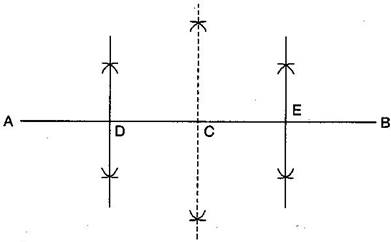(i) Draw a line segment AB = 12.8 cm

(ii) Draw the perpendicular bisector of {tex}\overline {{\text{AB}}} {/tex} which cuts it at C. Thus, C is the mid-point of {tex}\overline {{\text{AB}}} .{/tex}

(iii) Draw the perpendicular bisector of {tex}\overline {{\text{AC}}} {/tex} which cuts it at D. Thus D is the mid-point of .

(iv) Again, draw the perpendicular bisector of {tex}\overline {{\text{CB}}} {/tex} which cuts it at E. Thus, E is the mid-point of {tex}\overline {{\text{CB}}} {/tex}.

(v) Now, point C, D and E divide the line segment {tex}\overline {{\text{AB}}} {/tex} in the four equal parts.

(vi) By actual measurement, we find that

{tex}\overline {{\text{AD}}} = \overline {{\text{DC}}} = \overline {{\text{CE}}} = \overline {{\text{EB}}} = 3.2{/tex} cm

NCERT Solutions for Class 6 Maths Exercise 14.5

###### Question 5.With {tex}\overline {{\text{PQ}}} {/tex} of length 6.1 cm as diameter, draw a circle.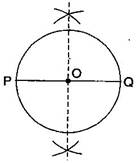(i) Draw a line segment {tex}\overline {{\text{PQ}}} {/tex} = 6.1 cm.

(ii) Draw the perpendicular bisector of PQ which cuts, it at O. Thus O is the mid-point of {tex}\overline {{\text{PQ}}} {/tex}.

Taking O as centre and OP or OQ as radius draw a circle where diameter is the line segment {tex}\overline {{\text{PQ}}} {/tex}.

###### Question 6.Draw a circle with centre C and radius 3.4 cm. Draw any chord {tex}\overline {{\text{AB}}} .{/tex} Construct the perpendicular bisector {tex}\overline {{\text{AB}}} {/tex} and examine if it passes through C.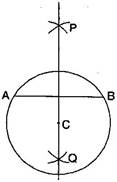(i) Draw a circle with centre C and radius 3.4 cm.

(ii) Draw any chord {tex}\overline {{\text{AB}}} .{/tex}

(iii) Taking A and B as centers and radius more than half of {tex}\overline {{\text{AB}}} ,{/tex} draw two arcs which cut each other at P and Q.

(iv) Join PQ. Then PQ is the perpendicular bisector of {tex}\overline {{\text{AB}}} .{/tex}

This perpendicular bisector of {tex}\overline {{\text{AB}}} {/tex} passes through the centre C of the circle.

NCERT Solutions for Class 6 Maths Exercise 14.5

###### Question 7.Repeat Question 6, if {tex}\overline {{\text{AB}}} {/tex} happens to be a diameter.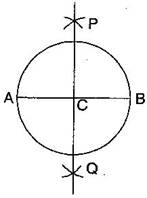(i) Draw a circle with centre C and radius 3.4 cm.

(ii) Draw its diameter {tex}\overline {{\text{AB}}} {/tex}.

(iii) Taking A and B as centers and radius more than half of it, draw two arcs which intersect each other at P and Q.

(iv) Join PQ. Then PQ is the perpendicular bisector of {tex}\overline {{\text{AB}}} {/tex}.

We observe that this perpendicular bisector of {tex}\overline {{\text{AB}}} {/tex} passes through the centre C of the circle.

NCERT Solutions for Class 6 Maths Exercise 14.5

###### Question 8.Draw a circle of radius 4 cm. Draw any two of its chords. Construct the perpendicular bisectors of these chords. Where do they meet?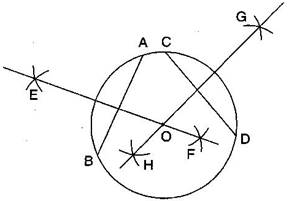(i) Draw the circle with O and radius 4 cm.

(ii) Draw any two chords {tex}\overline {{\text{AB}}} {/tex} and {tex}\overline {{\text{CD}}} {/tex} in this circle.

(iii) Taking A and B as centers and radius more than half AB, draw two arcs which intersect each other at E and F.

(iv) Join EF. Thus EF is the perpendicular bisector of chord {tex}\overline {{\text{CD}}} {/tex}.

(v) Similarly draw GH the perpendicular bisector of chord {tex}\overline {{\text{CD}}} {/tex}.

These two perpendicular bisectors meet at O, the centre of the circle.

NCERT Solutions for Class 6 Maths Exercise 14.5

###### Question 9.Draw any angle with vertex O. Take a point A on one of its arms and B on another such that OA = OB. Draw the perpendicular bisectors of {tex}\overline {{\text{OA}}} {/tex} and {tex}\overline {{\text{OB}}} .{/tex} Let them meet at P. Is PA = PB?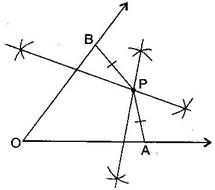(i) Draw any angle with vertex O.

(ii) Take a point A on one of its arms and B on another such that

(iii) Draw perpendicular bisector of {tex}\overline {{\text{OA}}} {/tex} and {tex}\overline {{\text{OB}}} .{/tex}

(iv) Let them meet at P. Join PA and PB.

With the help of divider, we check that {tex}\overline {{\text{PA}}} = \overline {{\text{PB}}} .{/tex}

## NCERT Solutions for Class 6 Maths Exercise 14.5

NCERT Solutions Class 6 Maths PDF (Download) Free from myCBSEguide app and myCBSEguide website. Ncert solution class 6 Maths includes text book solutions from Class 6 Maths Book . NCERT Solutions for CBSE Class 6 Maths have total 14 chapters. 6 Maths NCERT Solutions in PDF for free Download on our website. Ncert Maths class 6 solutions PDF and Maths ncert class 6 PDF solutions with latest modifications and as per the latest CBSE syllabus are only available in myCBSEguide.

## CBSE app for Students

To download NCERT Solutions for Class 6 Maths, Social Science Computer Science, Home Science, Hindi English, Maths Science do check myCBSEguide app or website. myCBSEguide provides sample papers with solution, test papers for chapter-wise practice, NCERT solutions, NCERT Exemplar solutions, quick revision notes for ready reference, CBSE guess papers and CBSE important question papers. Sample Paper all are made available through the best app for CBSE students and myCBSEguide website.Test Generator

Create question paper PDF and online tests with your own name & logo in minutes.myCBSEguide

Question Bank, Mock Tests, Exam Papers, NCERT Solutions, Sample Papers, Notes

### 3 thoughts on “NCERT Solutions for Class 6 Maths Exercise 14.5”

1. I’m telling draw angle abc=81 degree and draw its line of symmetry ok

2. Nice

3. Nice Question class 6th ncert ka liya aapna bhut kuch kiya mujhe bada acha laga thanx